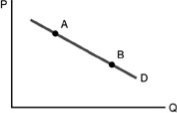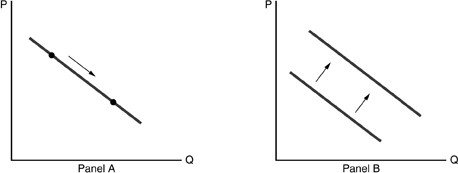• FAQ
• Contact/ Homework Answers / Economics / 81) An increase in demand occurs when A) we measure price
Not my Question
Flag Content

# Question : 81) An increase in demand occurs when A) we measure price

81) An increase in demand occurs when

A) we measure price in money price rather than real price.

B) we move up the demand curve.

C) the demand curve shifts to the right.

D) the demand curve shifts to the left.

82) A decrease in demand would be represented by

A) the price of a good going from \$3 to \$4.

B) an increase in the cost of resources used to produce the good.

C) a movement along the demand curve.

D) a shift of the demand curve to the left.

83) A normal good is one for which

A) demand increases as income increases.

B) demand increases as income decreases.

C) the demand curve is horizontal.

D) demand increases as the price of a substitute increases.

84) As John's income has increased, he has purchased fewer hamburgers. Hamburgers are

A) a normal good for John.

B) an inferior good for John.

C) not following the law of demand.

D) leading to a rightward shift in John's demand curve for hamburgers.85) Refer to the above figure. A movement from point A to point B indicates

A) an increase in demand.

B) a decrease in demand.

C) an increase in quantity demanded.

D) a decrease in quantity demanded.

86) Refer to the above figure. A movement from Point A to Point B is caused by

A) an increase in income.

B) an expectation of a decrease in the price of the good in that figure.

C) a decrease in the price of the good in that figure.

D) all of the above.87) Refer to the above figure. Which of the following statements is TRUE?

A) Panel A shows a decrease in demand and Panel B shows an increase in demand.

B) Both Panels A and B show an increase in demand.

C) Panel A shows a change in demand and Panel B shows a change in quantity demanded.

D) Panel A shows a change in quantity demanded and Panel B shows a change in demand.

88) Refer to the above figure. Which panel shows the effect of an increase in the price of a good on the demand curve of that good?

A) Panel A

B) Panel B

C) both panels

D) neither panel

89) A change in quantity demanded

A) is a shift of the demand curve.

B) is a movement along the demand curve.

C) can be either a shift or a movement along the demand curve.

D) is caused when there is a change in a ceteris paribus factor.

90) A change in any of the ceteris paribus conditions for demand leads to a

A) a good going from an inferior good to a normal good.

B) movement along the demand curve.

C) shift of the demand curve.

D) change in supply.

## Solution 5 (1 Ratings )

Solved
Economics 7 Months Ago 6 Views